﻿ 船用电动伺服舵机性能仿真研究
 舰船科学技术2016, Vol. 38Issue (6): 118-121PDF

Simulation research on shipboard electro-servo rudder actuator performance
XIAO Qing, XIE Jun-chao, HUA Jing
China Ship Development and Design Center, Wuhan 430064, China
Abstract: To reduce the traditional hydraulic steering noise and improve the maintainability of the ships, an electroservo rudder actuator program which mainly includes a DC torque electric motor and a harmonic gear was presented. The model of this actuator was established and the performance was simulated using MATLAB. The main factors which affected the performance of the rudder actuator were analyzed. The simulation results show the performance of this electro-servo rudder actuator can meet the steering requirements of the ship. The research results can be used to support the application of the electro-servo rudder actuator on ships.
Key words: electro-servo     rudder actuator     simulation
0 前 言

1 船用电动舵机方案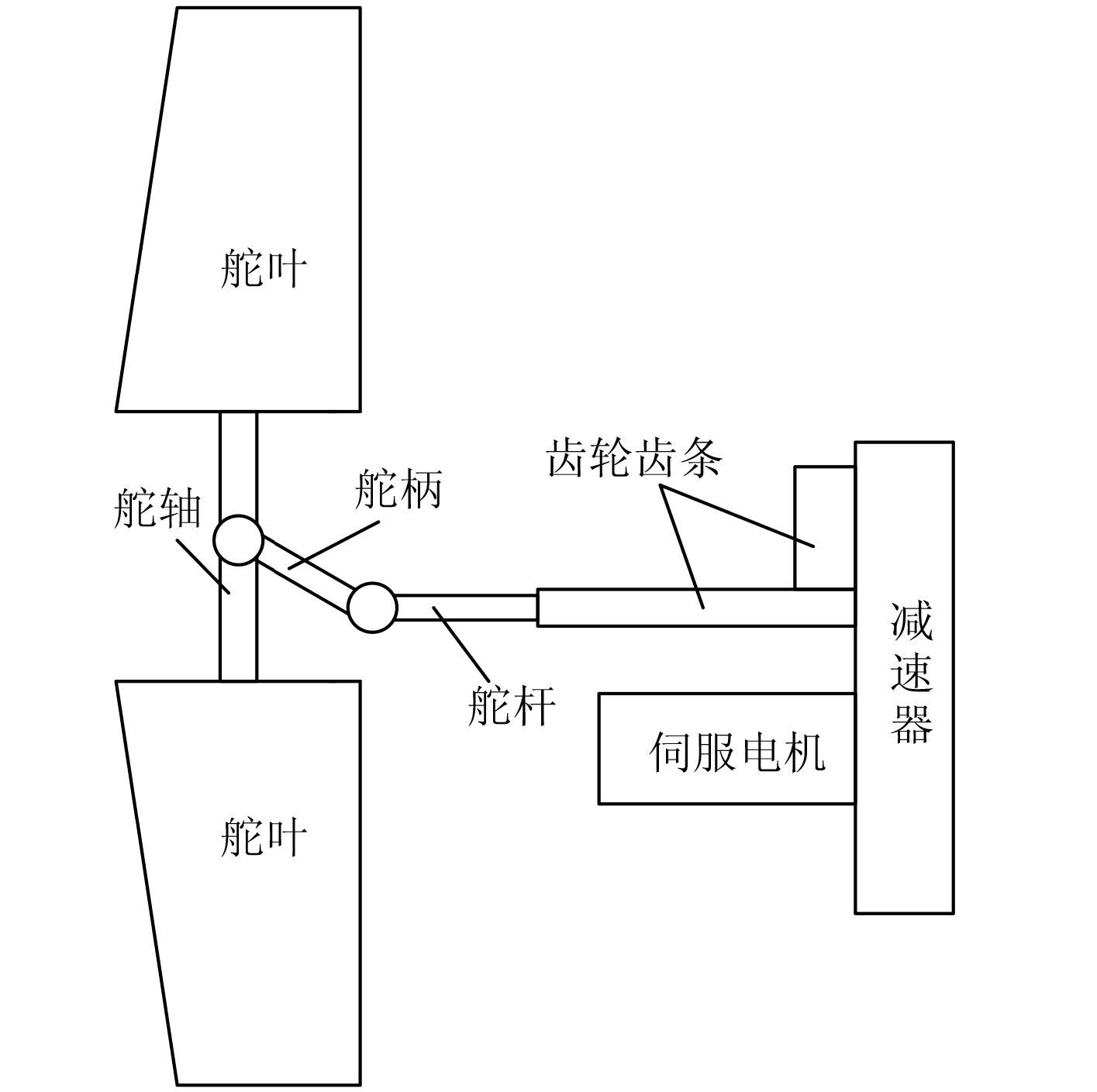图 1 电动伺服舵机示意图 Fig. 1 Schematic of the electro-servo rudder actuator表 1 伺服舵机主要性能指标 Tab.1 Main performance values of the servo rudder actuator表 2 电动伺服舵机的基本配置 Tab.2 Basic configuration of the electro-servo rudder actuator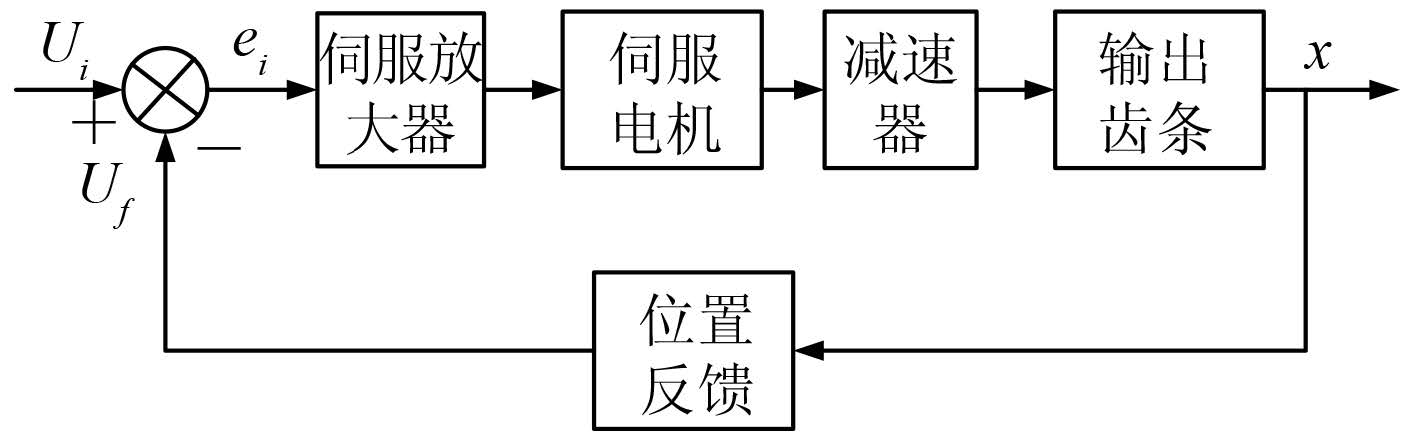图 2 电动伺服舵机控制框图 Fig. 2 Control diagram of the electro-servo rudder actuator
2 电动舵机性能仿真 2.1 仿真模型 2.1.1 伺服电机模型

 ${U_{{a}}} = R{I_{{a}}} + L\frac{{{\text{d}}{I_{{a}}}}}{{{\text{d}}t}} + E\text{，}$ (1)
 $E = {C_{{e}}}n\text{，}$ (2)
 ${T_{{{em}}}} - {T_{{L}}} = \frac{{G{D^2}{\text{d}}n}}{{375{\text{d}}t}}\text{，}$ (3)
 ${T_{{{em}}}} = {C_{{T}}}{I_{{a}}}\text{。}$ (4)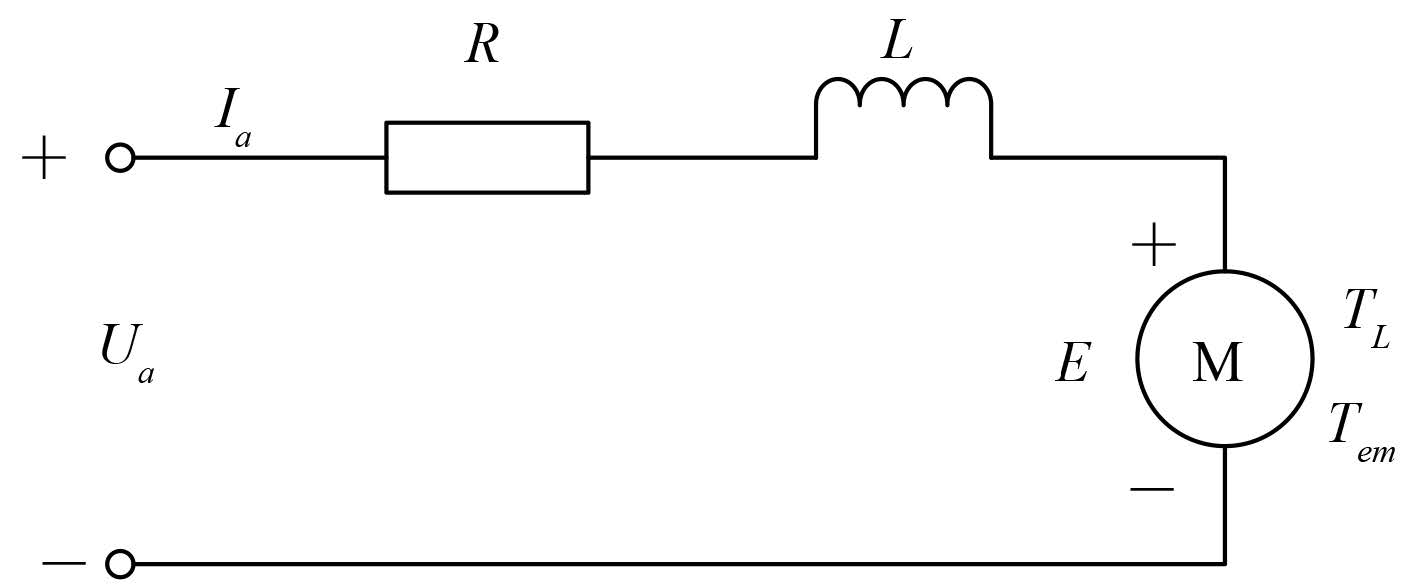图 3 伺服电机等效电路 Fig. 3 Equivalent circuit of the servo electric motor

TlTm 分别表示电气惯性与机械惯性的影响。代入式（1）~ 式（4）整理可得式（5）和式（6）：

 ${U_{{a}}} - E = R({I_{{a}}} + {T_{\text{l}}}\frac{{{\text{d}}{I_{{a}}}}}{{{\text{d}}t}})\text{，}$ (5)
 ${I_{{a}}} - {I_{{{aL}}}} = \frac{{{T_{{m}}}}}{R}\frac{{{\text{d}}E}}{{{\text{d}}t}}\text{，}$ (6)

 ${I_{{{aL}}}} = \frac{{{T_{{L}}}}}{{{C_{{T}}}}}\text{。}$ (7)

 $\frac{{{I_{{a}}}\left( {\text{s}} \right)}}{{U\left( {\text{s}} \right) - E\left( {\text{s}} \right)}} = \frac{{1/R}}{{{T_1}{\text{s}} + 1}}\text{，}$ (8)

 $\frac{{E\left( {\text{s}} \right)}}{{{I_a}\left( {\text{s}} \right) - {I_{{{aL}}}}\left( {\text{s}} \right)}} = \frac{R}{{{T_{{m}}}{\text{s}}}}\text{。}$ (9)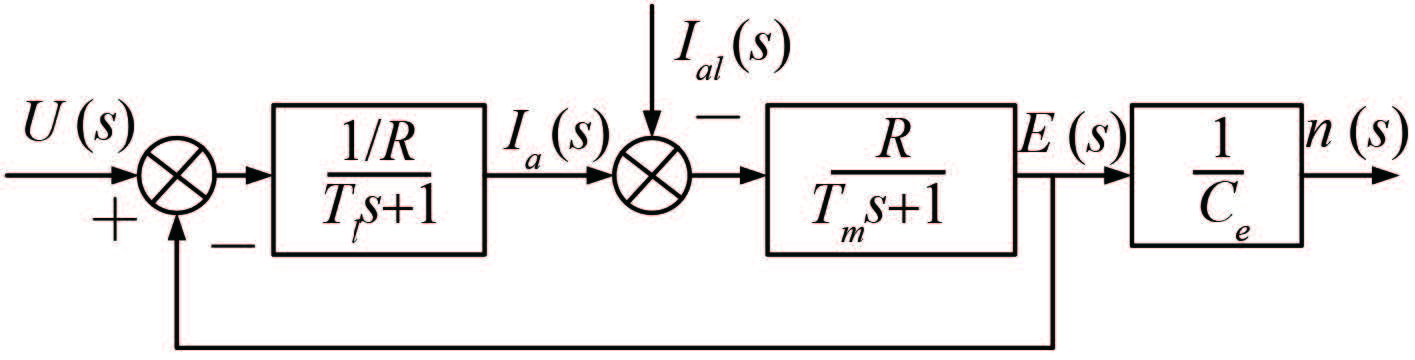图 4 伺服电机动态模型 Fig. 4 Dynamic model of the servo electric motor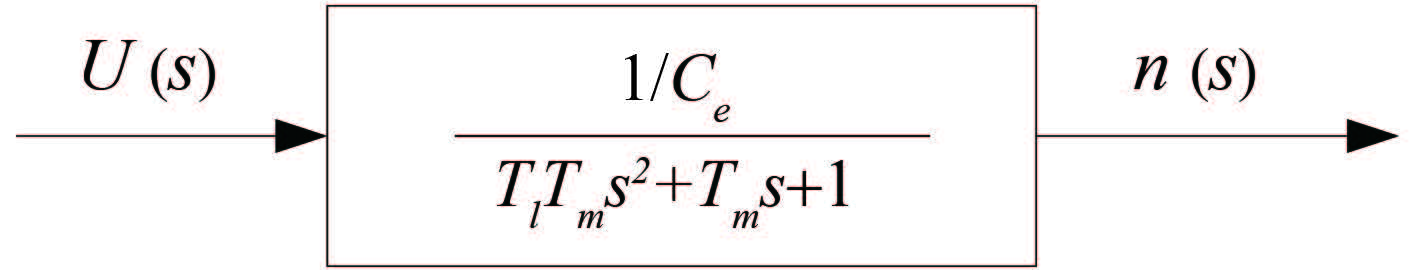图 5 伺服电机简化模型 Fig. 5 Simplified model of the servo electric motor
2.1.2 减速器模型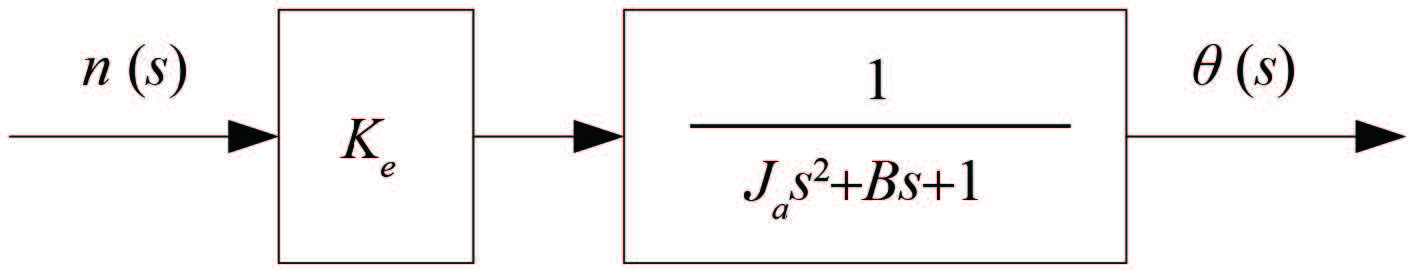图 6 谐波齿轮动态模型 Fig. 6 Dynamic model of the harmonic gear

 ${J_{{a}}} = {{\rm{J}}_{{x}}} + \frac{{{J_1}}}{{j_1^2}} + \frac{{{J_L}}}{{j_L^2}} + m\frac{{{{\rm{v}}^2}}}{{{\rm{\omega }}_{{x}}^2}}\text{。}$ (10)

2.1.3 负载模型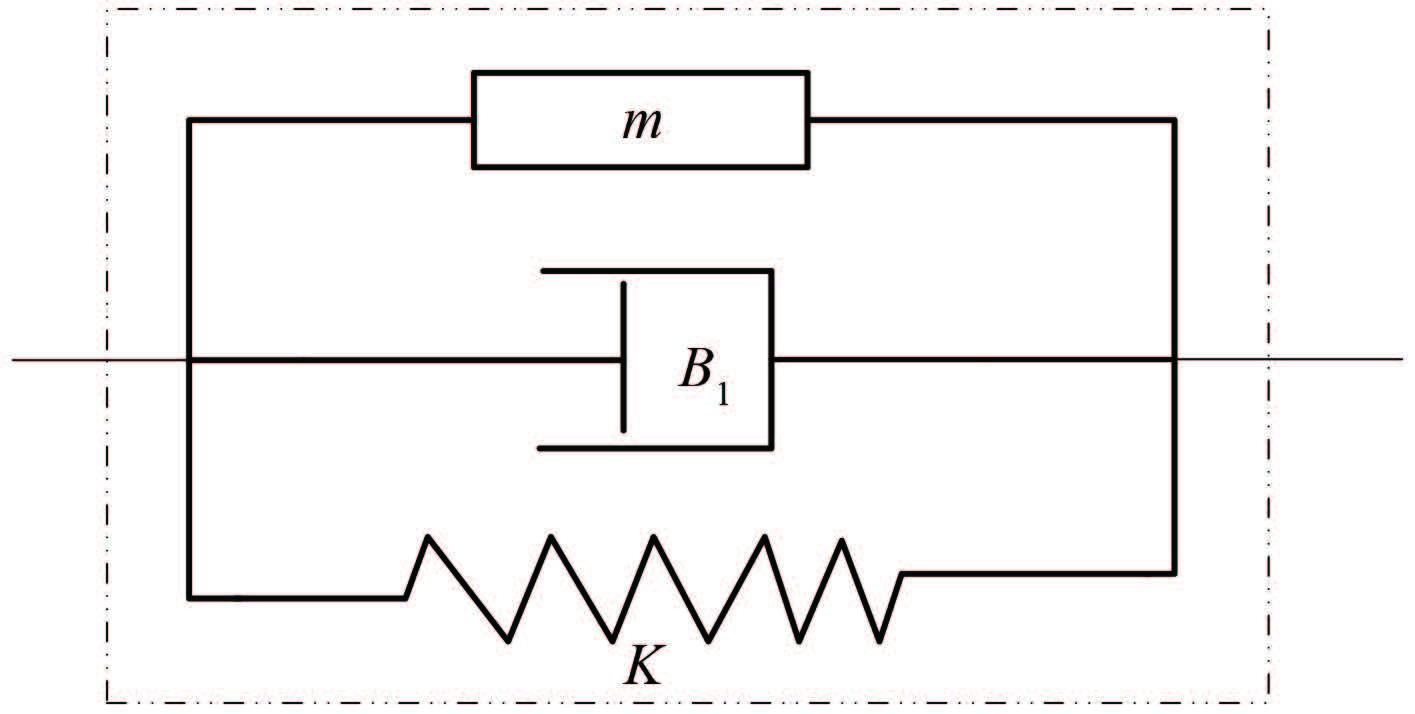图 7 负载等效模型 Fig. 7 Load equivalent model
2.2 仿真结果 2.2.1 仿真界面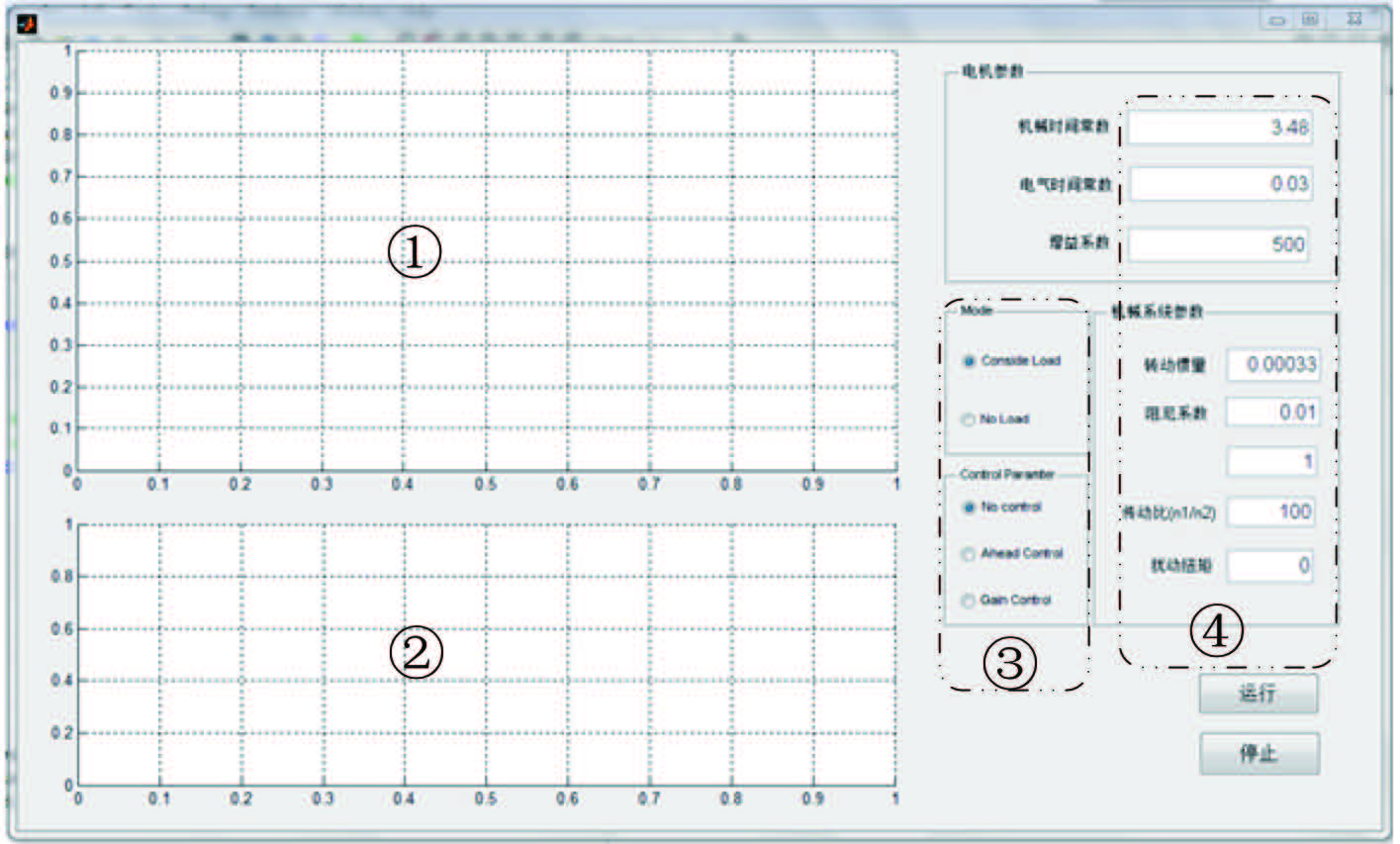图 8 仿真界面 Fig. 8 Simulation interface

2.2.2 仿真结果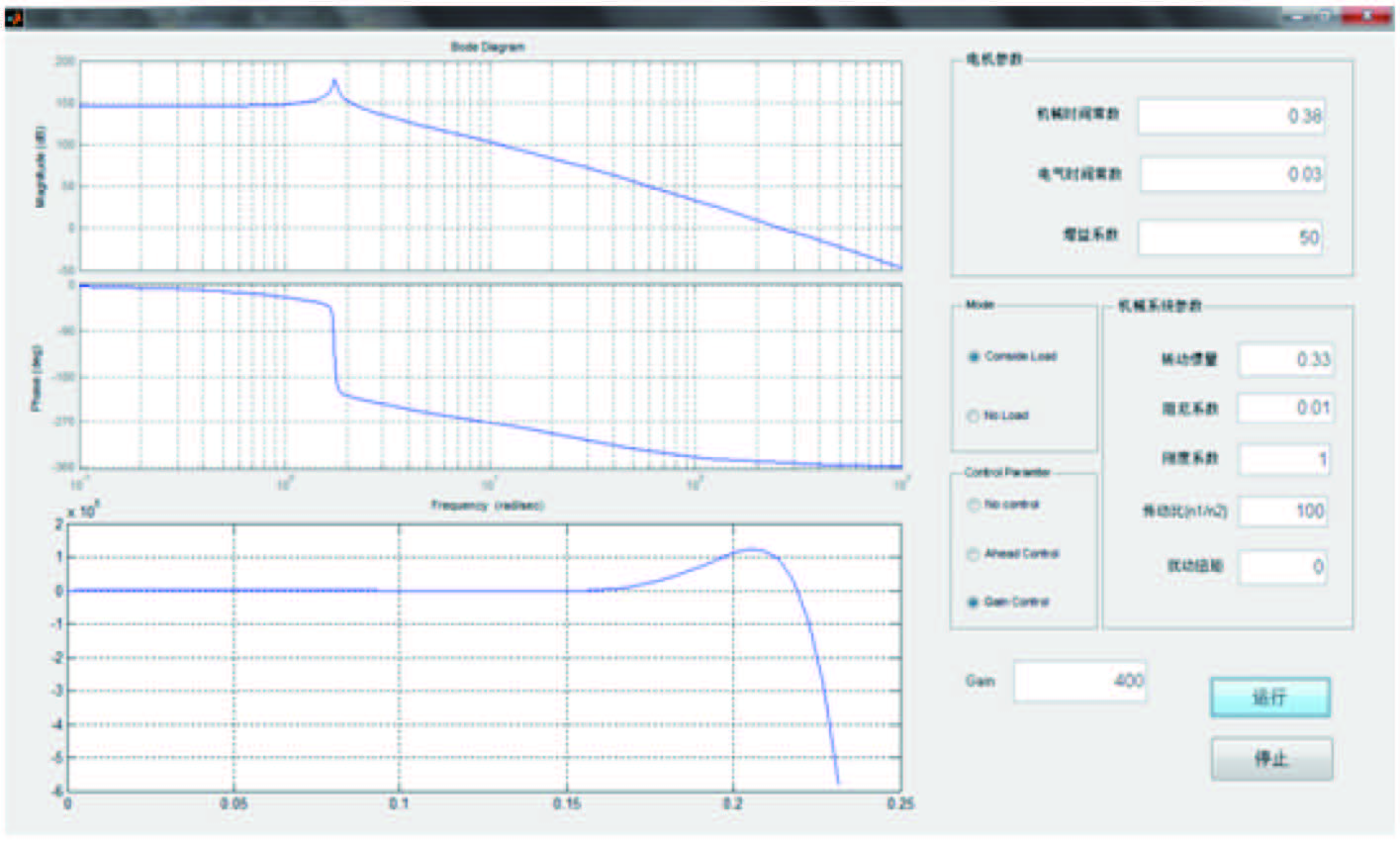图 9 带负载增益控制下的系统响应 Fig. 9 System response with load under gain control

1）影响系统稳定性的因素主要有阻尼、刚度等参数，在电动伺服舵机中，选用常用的直流力矩电机和谐波齿轮，系统的幅值裕度可达到 5 dB，相位裕度可达到 45°，满足稳定性的条件。

2）对于电动伺服舵机，影响精度的主要因素有伺服电机、谐波齿轮的刚度系数等，当刚度系数越大时，稳态误差越小，精度越高，对于直流力矩电机和谐波齿轮构成的电动伺服舵机，操舵舵角的稳态误差可控制在 0.2°（对应齿条直线位移为 0.2 mm），可满足舵系统这个大惯性系统的操作要求。

3）响应快是电动伺服系统的特点之一，影响其频响的主要因素有电机的电磁时间常数、机电时间常数、转动惯量、谐波齿轮的转动惯量等，根据本系统选择的元件类型，舵机的频响可大于 10 Hz。而负载为大惯性系统，其固有频率小于 1 Hz，因此电动伺服舵机的相应性能可满足操舵的需求。

3 结 语

  谢俊超, 肖清, 花靖. 船用新型电动舵机可行性研究[J]. 舰船科学技术 , 2013, 35 (10) :78–81. XIE Jun-chao, XIAO Qing, HUA Jing. Research on the new marine electro-mechanical actuator feasibility[J]. Ship Science and Technology , 2013, 35 (10) :78–81.  张阳阳, 邢继峰, 彭利坤, 等. 电动舵机用电动缸滚珠丝杠振动特性[J]. 舰船科学技术 , 2013, 35 (12) :29–31. ZHANG Yang-yang, XING Ji-feng, PENG Li-kun, et al. Ball screw vibration characteristic of electric cylinder used in electric helm[J]. Ship Science and Technology , 2013, 35 (12) :29–31.  王灿, 马瑞卿, 谭博, 等. 直流电动舵机伺服作动系统研制[J]. 微特电机 , 2008, 36 (10) :25–27. WANG Can, MA Rui-qing, TAN Bo, et al. Design of DC electric steering engine servo actuator system[J]. Small & Special Electrical Machines , 2008, 36 (10) :25–27.  李福瑞, 李擎, 夏嵩. 直流电动舵伺服系统设计与研究[J]. 北京信息科技大学学报 , 2010, 25 (1) :42–45. LI Fu-rui, LI Qing, XIA Song. Design and research of DC electric rudder servo system[J]. Journal of Beijing Information Science and Technology University , 2010, 25 (1) :42–45.  张新华. 完全双余度电动舵机系统的研究与设计[J]. 微电机 , 2006, 39 (2) :32–34. ZHANG Xin-hua. A research and design of entire dual redundancy electro-mechanical actuator[J]. Micromotors , 2006, 39 (2) :32–34.  董超奎, 段强. 微型无刷直流电动机在电动操舵系统中的应用[J]. 微电机 , 2006, 39 (6) :22–25. DONG Chao-kui, DUAN Qiang. Application of micro brushless DC motor on elector rudder system[J]. Micromotors , 2006, 39 (6) :22–25.  王建军, 杨宏源, 白会彦. 谐波齿轮传动在伺服机构中的应用与计算[J]. 战术导弹控制技术 , 2003 (1) :43–47. WANG Jian-jun, YANG Hong-yuan, BAI Hui-yan. Computation and application of harmonic gear drive in a servo system[J]. Control Technology of Tactical Missile , 2003 (1) :43–47.  霍学亮, 任光, 张均东, 等. 基于Simulink的船舶舵机系统的建模与动态仿真[J]. 大连海事大学学报 , 2007, 33 (S) :74–76. HUO Xue-liang, REN Guang, ZHANG Jun-dong, et al. Modeling and dynamic simulation of steering gear system of ship based on Simulink[J]. Journal of Dalian maritime university , 2007, 33 (S) :74–76.